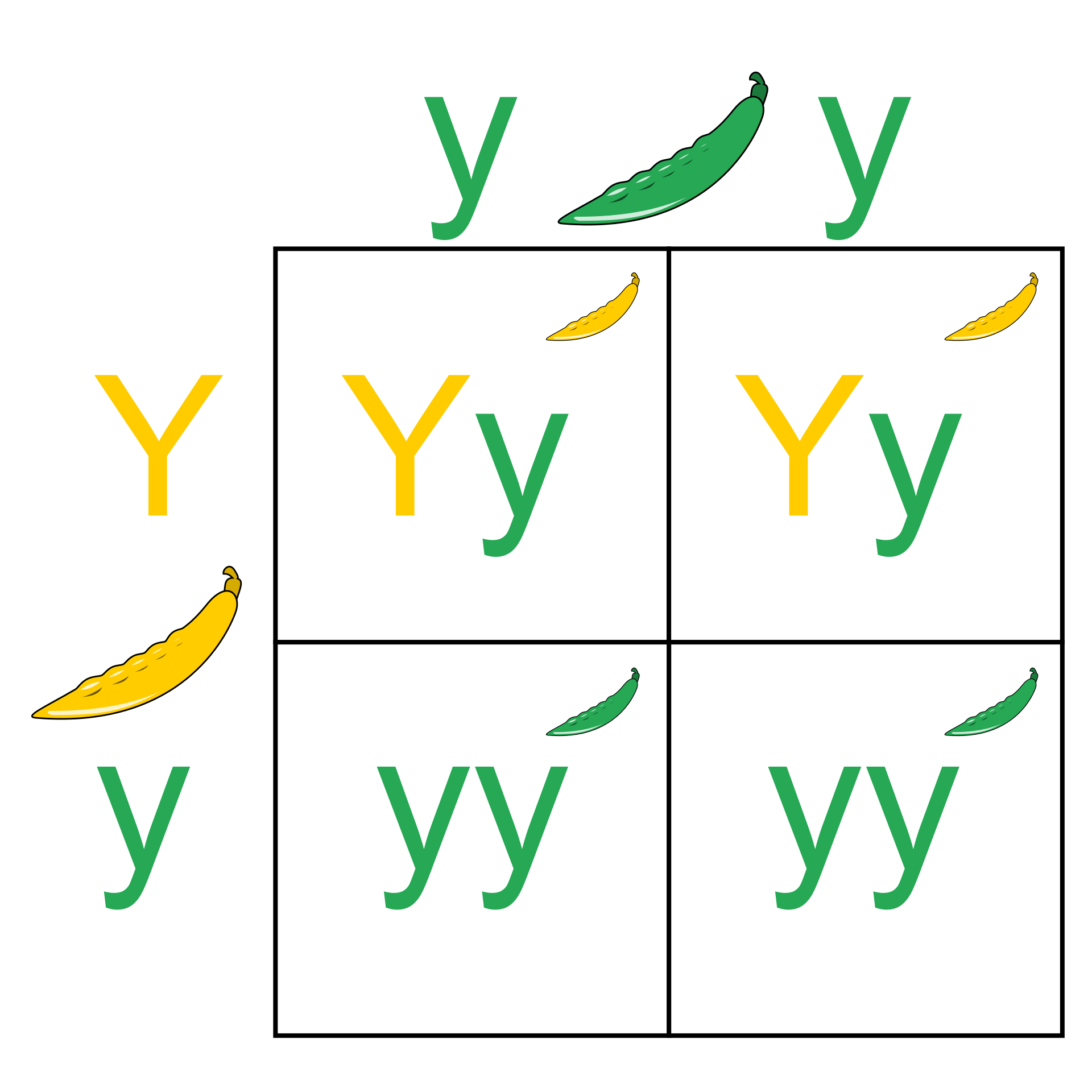# Basic Genetics

Biology Level 2The above picture shows punnet square for mono hybrid cross, involving the trait : colour of pea pod.

If we draw a punnet square for an $n$-hybrid cross ( A cross between two organisms, where $n$ traits are considered ), how many unit squares does it contain ?

If the answer is of the form $a^{bn}$ for positive integers $a,b > 1$ , enter $(a+b)$.

×